• 多边形和圆分开写，首先简单的就是判断是否在圆里面，如何判断一个坐标是否在圆形区域内，相信不用我说都知道，计算这个坐标点和圆心之间的距离，然后跟圆的半径进行比较，如果比半径大，就不在圆形区域内，如果小于...
怎么样判断一个坐标点在一个多边形区域内？包括规则多边形，不规则多边形，还有圆。。。
1 判断一个坐标是否在圆形区域内？
多边形和圆分开写，首先简单的就是判断是否在圆里面，如何判断一个坐标是否在圆形区域内，相信不用我说都知道，计算这个坐标点和圆心之间的距离，然后跟圆的半径进行比较，如果比半径大，就不在圆形区域内，如果小于等于圆的半径，则该坐标点在圆形区域内。
数学上的计算公式是这样的：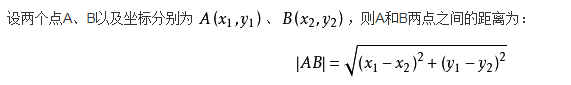代码采用谷歌地图计算距离的方式，应该算是比较精确。
private static double EARTH_RADIUS = 6378.137;

private static double rad(double d) {
return d * Math.PI / 180.0;
}

/**
* 通过经纬度获取距离(单位：米)
*
* @param lat1
* @param lng1
* @param lat2
* @param lng2
* @return
*/
public static double getDistance(double lat1, double lng1, double lat2,
double lng2) {
double s = 2 * Math.asin(Math.sqrt(Math.pow(Math.sin(a / 2), 2) +
s = Math.round(s * 10000d) / 10000d;
return s;
}

/**
* 判断一个点是否在圆形区域内
*/
public static boolean isInCircle(double lng1, double lat1, double lng2, double lat2, String radius) {
return getDistance(lat1, lng1, lat2, lng2) > Double.parseDouble(radius);
}
（如果使用Math.hypot()方法，计算(经纬度距离时)结果会有偏差）：
    double x = (lon1 - lon2) * PI * R * Math.cos(((lat1 + lat2) / 2) * PI / 180) / 180;
double y = (lat1 - lat2) * PI * R / 180;
double distance = Math.hypot(x, y);

2 判断一点是否在一个多边形区域内？

这里用到JAVA的关于坐标系和几何图形的一个类GeneralPath，使用这个类，结合传入的各顶点参数，画一个几何图形，并通过它自身的contains方法，判断一点是否在这个几何图形内。
也就是，通过JAVA已经封装好的方法，画一个几何多边形，判断一点是否在这个几何多边形里面。
代码里面也有注释：
/**
* 判断是否在多边形区域内
*
* @param pointLon
*            要判断的点的纵坐标
* @param pointLat
*            要判断的点的横坐标
* @param lon
*            区域各顶点的纵坐标数组
* @param lat
*            区域各顶点的横坐标数组
* @return
*/
public static boolean isInPolygon(double pointLon, double pointLat, double[] lon,
double[] lat) {
// 将要判断的横纵坐标组成一个点
Point2D.Double point = new Point2D.Double(pointLon, pointLat);
// 将区域各顶点的横纵坐标放到一个点集合里面
List<Point2D.Double> pointList = new ArrayList<Point2D.Double>();
double polygonPoint_x = 0.0, polygonPoint_y = 0.0;
for (int i = 0; i < lon.length; i++) {
polygonPoint_x = lon[i];
polygonPoint_y = lat[i];
Point2D.Double polygonPoint = new Point2D.Double(polygonPoint_x, polygonPoint_y);
}
return check(point, pointList);
}

/**
* 一个点是否在多边形内
*
* @param point
*            要判断的点的横纵坐标
* @param polygon
*            组成的顶点坐标集合
* @return
*/
private static boolean check(Point2D.Double point, List<Point2D.Double> polygon) {
java.awt.geom.GeneralPath peneralPath = new java.awt.geom.GeneralPath();

Point2D.Double first = polygon.get(0);
// 通过移动到指定坐标（以双精度指定），将一个点添加到路径中
peneralPath.moveTo(first.x, first.y);
polygon.remove(0);
for (Point2D.Double d : polygon) {
// 通过绘制一条从当前坐标到新指定坐标（以双精度指定）的直线，将一个点添加到路径中。
peneralPath.lineTo(d.x, d.y);
}
// 将几何多边形封闭
peneralPath.lineTo(first.x, first.y);
peneralPath.closePath();
// 测试指定的 Point2D 是否在 Shape 的边界内。
return peneralPath.contains(point);
}
有没有感觉很方便很简单。。。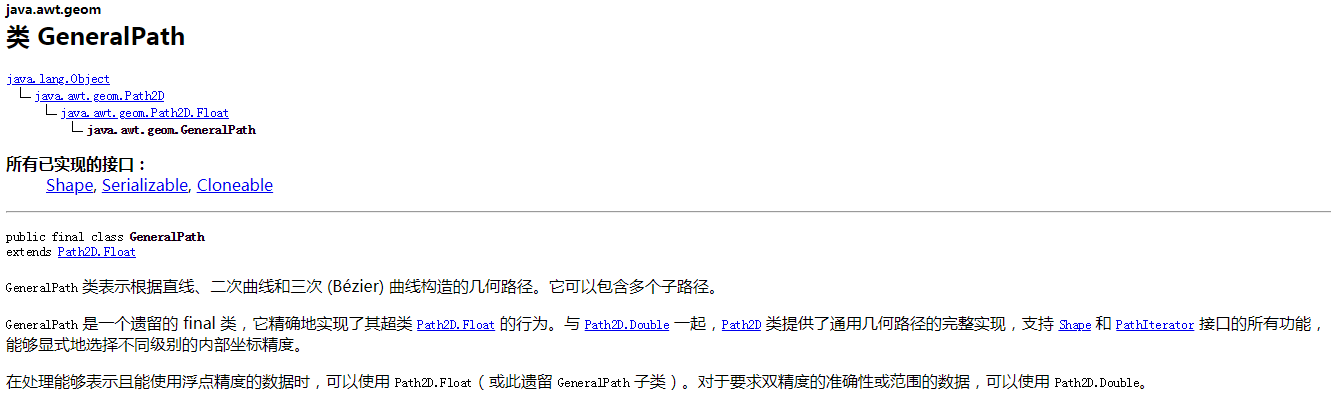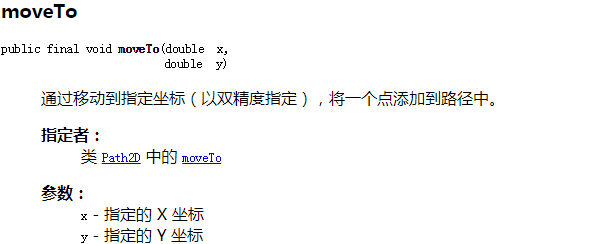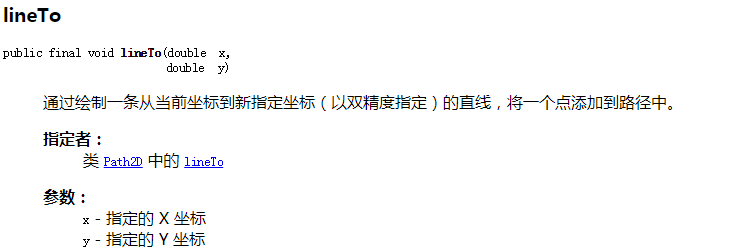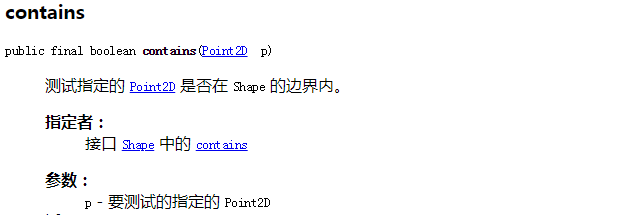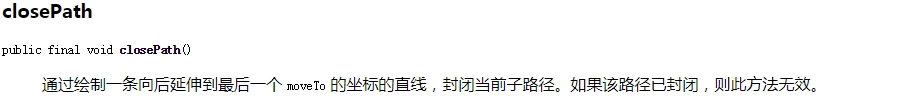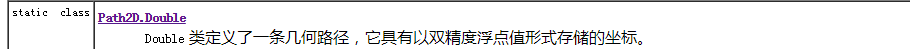以上是用到的各方法介绍。。。
3 当然解决问题的方法肯定不止一种。这里还有一种比较简单的判断一点是否在一个多边形区域内的方法
先将横纵坐标数组的横坐标最大最小值，纵坐标的最大最小值，求出来，需要判断的一点大于横纵坐标的最大值或者小于横纵坐标的最小值，也就是粗略的计算一下，如果这个条件不满足的话，就不用往下计算了，直接不在指定的区域里面。
也就是：
/**
* 判断该地理坐标是否在最大范围区域内
*
* @param pointLon
*            要判断的点的纵坐标
* @param pointLat
*            要判断的点的横坐标
* @param lon
*            指定区域的纵坐标组成的数组
* @param lat
*            指定区域的横坐标组成的数组
* @return
*/
private static boolean isInMaxArea(double pointLon, double pointLat, double[] lon,
double[] lat) {

// 获取区域横纵坐标最大值和最小值
double temp = 0.0;
for (int i = 0; i < lon.length; i++) {
for (int j = 0; j < lon.length - i - 1; j++) {
if (lon[j] > lon[j + 1]) {
temp = lon[j];
lon[j] = lon[j + 1];
lon[j + 1] = temp;
}
}
}
for (int i = 0; i < lat.length; i++) {
for (int j = 0; j < lat.length - i - 1; j++) {
if (lat[j] > lat[j + 1]) {
temp = lat[j];
lat[j] = lat[j + 1];
lat[j + 1] = temp;
}
}
}

// 如果在最值组成的区域外，那肯定不在重点区域内
return (pointLon < lon || pointLon > lon[lon.length - 1] || pointLat < lat
|| pointLat > lat[lat.length - 1]);
}
如果通过了上面的判断，可以进行接下来的算法判断了
用到了两点间的斜率公式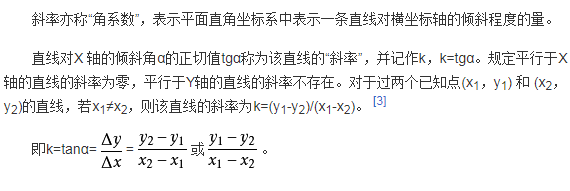这个方法就是，通过一点，画一条线，这条线与多边形相交，如果相交点数位奇数，就在区域内，如果为偶数，就不在区域内
代码：
/**
* 判断坐标是否在重点区域内
*
* @param pointLon
*            要判断的点的纵坐标
* @param pointLat
*            要判断的点的横坐标
* @param lon
*            指定区域的纵坐标组成的数组
* @param lat
*            指定区域的横坐标组成的数组
* @return
*/
private static boolean isInAccurateArea(double pointLon, double pointLat, double[] lon,
double[] lat) {
// 代表有几个点
int vertexNum = lon.length;
boolean result = false;

for (int i = 0, j = vertexNum - 1; i < vertexNum; j = i++) {
// 满足条件，与多边形相交一次，result布尔值取反一次，奇数个则在区域内
if ((lon[i] > pointLon) != (lon[j] > pointLon)
&& (pointLat < (lat[j] - lat[i]) * (pointLon - lon[i]) / (lon[j] - lon[i])
+ lat[i])) {
result = !result;
}
}
return result;
}
好了，就这些了，哪里不对欢迎指教。。。有问题也可以探讨。
展开全文JAVA
• 学习过程中遇到了需要判断坐标点是否在区域内的需求，所以发现了这篇文章，特地转载过来

转载自 http://blog.csdn.net/c1481118216/article/details/52661934

首先提供一个百度地图API官方的GeoUtils.js   直接调用即可
  /**
* @fileoverview GeoUtils类提供若干几何算法，用来帮助用户判断点与矩形、
* 圆形、多边形线、多边形面的关系,并提供计算折线长度和多边形的面积的公式。
* 主入口类是<a href="symbols/BMapLib.GeoUtils.html">GeoUtils</a>，
* 基于Baidu Map API 1.2。
*
* @author Baidu Map Api Group
* @version 1.2
*/

/**
* @namespace BMap的所有library类均放在BMapLib命名空间下
*/
var BMapLib = window.BMapLib = BMapLib || {};
(function() {

/**
* 地球半径
*/

/**
* @exports GeoUtils as BMapLib.GeoUtils
*/
var GeoUtils =
/**
* GeoUtils类，静态类，勿需实例化即可使用
* @class GeoUtils类的<b>入口</b>。
* 该类提供的都是静态方法，勿需实例化即可使用。
*/
BMapLib.GeoUtils = function(){

}

/**
* 判断点是否在矩形内
* @param {Point} point 点对象
* @param {Bounds} bounds 矩形边界对象
* @returns {Boolean} 点在矩形内返回true,否则返回false
*/
GeoUtils.isPointInRect = function(point, bounds){
//检查类型是否正确
if (!(point instanceof BMap.Point) ||
!(bounds instanceof BMap.Bounds)) {
return false;
}
var sw = bounds.getSouthWest(); //西南脚点
var ne = bounds.getNorthEast(); //东北脚点
return (point.lng >= sw.lng && point.lng <= ne.lng && point.lat >= sw.lat && point.lat <= ne.lat);
}

/**
* 判断点是否在圆形内
* @param {Point} point 点对象
* @param {Circle} circle 圆形对象
* @returns {Boolean} 点在圆形内返回true,否则返回false
*/
GeoUtils.isPointInCircle = function(point, circle){

//检查类型是否正确
if (!(point instanceof BMap.Point) ||
!(circle instanceof BMap.Circle)) {
return false;
}
//point与圆心距离小于圆形半径，则点在圆内，否则在圆外
var c = circle.getCenter();

var dis = GeoUtils.getDistance(point, c);
if(dis <= r){
return true;
} else {
return false;
}
}

/**
* 判断点是否在折线上
* @param {Point} point 点对象
* @param {Polyline} polyline 折线对象
* @returns {Boolean} 点在折线上返回true,否则返回false
*/
GeoUtils.isPointOnPolyline = function(point, polyline){
//检查类型
if(!(point instanceof BMap.Point) ||
!(polyline instanceof BMap.Polyline)){
return false;
}

//首先判断点是否在线的外包矩形内，如果在，则进一步判断，否则返回false
var lineBounds = polyline.getBounds();
if(!this.isPointInRect(point, lineBounds)){
return false;
}

//判断点是否在线段上，设点为Q，线段为P1P2 ，
//判断点Q在该线段上的依据是：( Q - P1 ) × ( P2 - P1 ) = 0，且 Q 在以 P1，P2为对角顶点的矩形内
var pts = polyline.getPath();
for(var i = 0; i < pts.length - 1; i++){
var curPt = pts[i];
var nextPt = pts[i + 1];
//首先判断point是否在curPt和nextPt之间，即：此判断该点是否在该线段的外包矩形内
if (point.lng >= Math.min(curPt.lng, nextPt.lng) && point.lng <= Math.max(curPt.lng, nextPt.lng) &&
point.lat >= Math.min(curPt.lat, nextPt.lat) && point.lat <= Math.max(curPt.lat, nextPt.lat)){
//判断点是否在直线上公式
var precision = (curPt.lng - point.lng) * (nextPt.lat - point.lat) -
(nextPt.lng - point.lng) * (curPt.lat - point.lat);
if(precision < 2e-10 && precision > -2e-10){//实质判断是否接近0
return true;
}
}
}

return false;
}

/**
* 判断点是否多边形内
* @param {Point} point 点对象
* @param {Polyline} polygon 多边形对象
* @returns {Boolean} 点在多边形内返回true,否则返回false
*/
GeoUtils.isPointInPolygon = function(point, polygon){

//检查类型
if(!(point instanceof BMap.Point) ||
!(polygon instanceof BMap.Polygon)){

return false;
}

//首先判断点是否在多边形的外包矩形内，如果在，则进一步判断，否则返回false

var polygonBounds = polygon.getBounds();
if(!this.isPointInRect(point, polygonBounds)){
return false;
}
var pts = polygon.getPath();//获取多边形点

//下述代码来源：http://paulbourke.net/geometry/insidepoly/，进行了部分修改
//基本思想是利用射线法，计算射线与多边形各边的交点，如果是偶数，则点在多边形外，否则
//在多边形内。还会考虑一些特殊情况，如点在多边形顶点上，点在多边形边上等特殊情况。

var N = pts.length;
var boundOrVertex = true; //如果点位于多边形的顶点或边上，也算做点在多边形内，直接返回true
var intersectCount = 0;//cross points count of x
var precision = 2e-10; //浮点类型计算时候与0比较时候的容差
var p1, p2;//neighbour bound vertices
var p = point; //测试点

p1 = pts;//left vertex
for(var i = 1; i <= N; ++i){//check all rays
if(p.equals(p1)){
return boundOrVertex;//p is an vertex
}

p2 = pts[i % N];//right vertex
if(p.lat < Math.min(p1.lat, p2.lat) || p.lat > Math.max(p1.lat, p2.lat)){//ray is outside of our interests
p1 = p2;
continue;//next ray left point
}

if(p.lat > Math.min(p1.lat, p2.lat) && p.lat < Math.max(p1.lat, p2.lat)){//ray is crossing over by the algorithm (common part of)
if(p.lng <= Math.max(p1.lng, p2.lng)){//x is before of ray
if(p1.lat == p2.lat && p.lng >= Math.min(p1.lng, p2.lng)){//overlies on a horizontal ray
return boundOrVertex;
}

if(p1.lng == p2.lng){//ray is vertical
if(p1.lng == p.lng){//overlies on a vertical ray
return boundOrVertex;
}else{//before ray
++intersectCount;
}
}else{//cross point on the left side
var xinters = (p.lat - p1.lat) * (p2.lng - p1.lng) / (p2.lat - p1.lat) + p1.lng;//cross point of lng
if(Math.abs(p.lng - xinters) < precision){//overlies on a ray
return boundOrVertex;
}

if(p.lng < xinters){//before ray
++intersectCount;
}
}
}
}else{//special case when ray is crossing through the vertex
if(p.lat == p2.lat && p.lng <= p2.lng){//p crossing over p2
var p3 = pts[(i+1) % N]; //next vertex
if(p.lat >= Math.min(p1.lat, p3.lat) && p.lat <= Math.max(p1.lat, p3.lat)){//p.lat lies between p1.lat & p3.lat
++intersectCount;
}else{
intersectCount += 2;
}
}
}
p1 = p2;//next ray left point
}
if(intersectCount % 2 == 0){//偶数在多边形外
return false;
} else { //奇数在多边形内
return true;
}
}

/**
* 将度转化为弧度
* @param {degree} Number 度
* @returns {Number} 弧度
*/
return Math.PI * degree/180;
}

/**
* 将弧度转化为度
* @returns {Number} 度
*/
return (180 * rad) / Math.PI;
}

/**
* 将v值限定在a,b之间，纬度使用
*/
function _getRange(v, a, b){
if(a != null){
v = Math.max(v, a);
}
if(b != null){
v = Math.min(v, b);
}
return v;
}

/**
* 将v值限定在a,b之间，经度使用
*/
function _getLoop(v, a, b){
while( v > b){
v -= b - a
}
while(v < a){
v += b - a
}
return v;
}

/**
* 计算两点之间的距离,两点坐标必须为经纬度
* @param {point1} Point 点对象
* @param {point2} Point 点对象
* @returns {Number} 两点之间距离，单位为米
*/
GeoUtils.getDistance = function(point1, point2){
//判断类型
if(!(point1 instanceof BMap.Point) ||
!(point2 instanceof BMap.Point)){
return 0;
}

point1.lng = _getLoop(point1.lng, -180, 180);
point1.lat = _getRange(point1.lat, -74, 74);
point2.lng = _getLoop(point2.lng, -180, 180);
point2.lat = _getRange(point2.lat, -74, 74);

var x1, x2, y1, y2;

return EARTHRADIUS * Math.acos((Math.sin(y1) * Math.sin(y2) + Math.cos(y1) * Math.cos(y2) * Math.cos(x2 - x1)));
}

/**
* 计算折线或者点数组的长度
* @param {Polyline|Array<Point>} polyline 折线对象或者点数组
* @returns {Number} 折线或点数组对应的长度
*/
GeoUtils.getPolylineDistance = function(polyline){
//检查类型
if(polyline instanceof BMap.Polyline ||
polyline instanceof Array){
//将polyline统一为数组
var pts;
if(polyline instanceof BMap.Polyline){
pts = polyline.getPath();
} else {
pts = polyline;
}

if(pts.length < 2){//小于2个点，返回0
return 0;
}

//遍历所有线段将其相加，计算整条线段的长度
var totalDis = 0;
for(var i =0; i < pts.length - 1; i++){
var curPt = pts[i];
var nextPt = pts[i + 1]
var dis = GeoUtils.getDistance(curPt, nextPt);
totalDis += dis;
}

} else {
return 0;
}
}

/**
* 计算多边形面或点数组构建图形的面积,注意：坐标类型只能是经纬度，且不适合计算自相交多边形的面积
* @param {Polygon|Array<Point>} polygon 多边形面对象或者点数组
* @returns {Number} 多边形面或点数组构成图形的面积
*/
GeoUtils.getPolygonArea = function(polygon){
//检查类型
if(!(polygon instanceof BMap.Polygon) &&
!(polygon instanceof Array)){
return 0;
}
var pts;
if(polygon instanceof BMap.Polygon){
pts = polygon.getPath();
}else{
pts = polygon;
}

if(pts.length < 3){//小于3个顶点，不能构建面
return 0;
}

var totalArea = 0;//初始化总面积
var LowX = 0.0;
var LowY = 0.0;
var MiddleX = 0.0;
var MiddleY = 0.0;
var HighX = 0.0;
var HighY = 0.0;
var AM = 0.0;
var BM = 0.0;
var CM = 0.0;
var AL = 0.0;
var BL = 0.0;
var CL = 0.0;
var AH = 0.0;
var BH = 0.0;
var CH = 0.0;
var CoefficientL = 0.0;
var CoefficientH = 0.0;
var ALtangent = 0.0;
var BLtangent = 0.0;
var CLtangent = 0.0;
var AHtangent = 0.0;
var BHtangent = 0.0;
var CHtangent = 0.0;
var ANormalLine = 0.0;
var BNormalLine = 0.0;
var CNormalLine = 0.0;
var OrientationValue = 0.0;
var AngleCos = 0.0;
var Sum1 = 0.0;
var Sum2 = 0.0;
var Count2 = 0;
var Count1 = 0;
var Sum = 0.0;
var Count = pts.length;
for (var i = 0; i < Count; i++) {
if (i == 0) {
LowX = pts[Count - 1].lng * Math.PI / 180;
LowY = pts[Count - 1].lat * Math.PI / 180;
MiddleX = pts.lng * Math.PI / 180;
MiddleY = pts.lat * Math.PI / 180;
HighX = pts.lng * Math.PI / 180;
HighY = pts.lat * Math.PI / 180;
}
else if (i == Count - 1) {
LowX = pts[Count - 2].lng * Math.PI / 180;
LowY = pts[Count - 2].lat * Math.PI / 180;
MiddleX = pts[Count - 1].lng * Math.PI / 180;
MiddleY = pts[Count - 1].lat * Math.PI / 180;
HighX = pts.lng * Math.PI / 180;
HighY = pts.lat * Math.PI / 180;
}
else {
LowX = pts[i - 1].lng * Math.PI / 180;
LowY = pts[i - 1].lat * Math.PI / 180;
MiddleX = pts[i].lng * Math.PI / 180;
MiddleY = pts[i].lat * Math.PI / 180;
HighX = pts[i + 1].lng * Math.PI / 180;
HighY = pts[i + 1].lat * Math.PI / 180;
}
AM = Math.cos(MiddleY) * Math.cos(MiddleX);
BM = Math.cos(MiddleY) * Math.sin(MiddleX);
CM = Math.sin(MiddleY);
AL = Math.cos(LowY) * Math.cos(LowX);
BL = Math.cos(LowY) * Math.sin(LowX);
CL = Math.sin(LowY);
AH = Math.cos(HighY) * Math.cos(HighX);
BH = Math.cos(HighY) * Math.sin(HighX);
CH = Math.sin(HighY);
CoefficientL = (AM * AM + BM * BM + CM * CM) / (AM * AL + BM * BL + CM * CL);
CoefficientH = (AM * AM + BM * BM + CM * CM) / (AM * AH + BM * BH + CM * CH);
ALtangent = CoefficientL * AL - AM;
BLtangent = CoefficientL * BL - BM;
CLtangent = CoefficientL * CL - CM;
AHtangent = CoefficientH * AH - AM;
BHtangent = CoefficientH * BH - BM;
CHtangent = CoefficientH * CH - CM;
AngleCos = (AHtangent * ALtangent + BHtangent * BLtangent + CHtangent * CLtangent) / (Math.sqrt(AHtangent * AHtangent + BHtangent * BHtangent + CHtangent * CHtangent) * Math.sqrt(ALtangent * ALtangent + BLtangent * BLtangent + CLtangent * CLtangent));
AngleCos = Math.acos(AngleCos);
ANormalLine = BHtangent * CLtangent - CHtangent * BLtangent;
BNormalLine = 0 - (AHtangent * CLtangent - CHtangent * ALtangent);
CNormalLine = AHtangent * BLtangent - BHtangent * ALtangent;
if (AM != 0)
OrientationValue = ANormalLine / AM;
else if (BM != 0)
OrientationValue = BNormalLine / BM;
else
OrientationValue = CNormalLine / CM;
if (OrientationValue > 0) {
Sum1 += AngleCos;
Count1++;
}
else {
Sum2 += AngleCos;
Count2++;
}
}
var tempSum1, tempSum2;
tempSum1 = Sum1 + (2 * Math.PI * Count2 - Sum2);
tempSum2 = (2 * Math.PI * Count1 - Sum1) + Sum2;
if (Sum1 > Sum2) {
if ((tempSum1 - (Count - 2) * Math.PI) < 1)
Sum = tempSum1;
else
Sum = tempSum2;
}
else {
if ((tempSum2 - (Count - 2) * Math.PI) < 1)
Sum = tempSum2;
else
Sum = tempSum1;
}
totalArea = (Sum - (Count - 2) * Math.PI) * Radius * Radius;

}

})();//闭包结束
123456789101112131415161718192021222324252627282930313233343536373839404142434445464748495051525354555657585960616263646566676869707172737475767778798081828384858687888990919293949596979899100101102103104105106107108109110111112113114115116117118119120121122123124125126127128129130131132133134135136137138139140141142143144145146147148149150151152153154155156157158159160161162163164165166167168169170171172173174175176177178179180181182183184185186187188189190191192193194195196197198199200201202203204205206207208209210211212213214215216217218219220221222223224225226227228229230231232233234235236237238239240241242243244245246247248249250251252253254255256257258259260261262263264265266267268269270271272273274275276277278279280281282283284285286287288289290291292293294295296297298299300301302303304305306307308309310311312313314315316317318319320321322323324325326327328329330331332333334335336337338339340341342343344345346347348349350351352353354355356357358359360361362363364365366367368369370371372373374375376377378379380381382383384385386387388389390391392393394395396397398399400401402403404405406407408409410411412413414415416417418419420421422423424425426427428429430431432433434435436437438439440441442443444445446447448449450451452453454455456
123456789101112131415161718192021222324252627282930313233343536373839404142434445464748495051525354555657585960616263646566676869707172737475767778798081828384858687888990919293949596979899100101102103104105106107108109110111112113114115116117118119120121122123124125126127128129130131132133134135136137138139140141142143144145146147148149150151152153154155156157158159160161162163164165166167168169170171172173174175176177178179180181182183184185186187188189190191192193194195196197198199200201202203204205206207208209210211212213214215216217218219220221222223224225226227228229230231232233234235236237238239240241242243244245246247248249250251252253254255256257258259260261262263264265266267268269270271272273274275276277278279280281282283284285286287288289290291292293294295296297298299300301302303304305306307308309310311312313314315316317318319320321322323324325326327328329330331332333334335336337338339340341342343344345346347348349350351352353354355356357358359360361362363364365366367368369370371372373374375376377378379380381382383384385386387388389390391392393394395396397398399400401402403404405406407408409410411412413414415416417418419420421422423424425426427428429430431432433434435436437438439440441442443444445446447448449450451452453454455456
使用方法核心代码：
//创建一个圆(坐标自己填)
var circle = new BMap.Circle(new BMap.Point(112.595384,26.904631),1000,{fillColor:"blue", strokeWeight: 1 ,fillOpacity: 0.3, strokeOpacity: 0.3});
//创建一个点(坐标自己填)
var point = new BMap.Point(112.595384,26.904631);    // 创建点坐标
//判断点是否在圆形区域内
if(BMapLib.GeoUtils.isPointInCircle(point,circle)){
}else
{
}
1234567891011
1234567891011
编码中遇到的问题：
要注意Point和Circle 都必须是:BMap.Point和BMap.Bounds对象
Tip：
官方js链接： http://api.map.baidu.com/library/GeoUtils/1.2/docs/symbols/src/BMapLib_GeoUtils.js.html演示地址：圆形：http://www.ltbetter.com:8080/BMap/MapTest5.html  多边形：http://www.ltbetter.com:8080/BMap/MapTest6.html多边形，矩形类似，官方api都有相应的方法
源代码 圆形：
<!DOCTYPE html>
<html>
<meta http-equiv="Content-Type" content="text/html; charset=utf-8" />
<meta name="viewport" content="initial-scale=1.0, user-scalable=no" />
<style type="text/css">
body, html,#allmap {width: 100%;height: 100%;overflow: hidden;margin:0;font-family:"微软雅黑";}
</style>
<script type="text/javascript" src="http://api.map.baidu.com/api?v=2.0&ak=ZwQAeZO1fncN63zUmuYeGU7CLU0mpfdk"></script>
<script type="text/javascript" src="GeoUtil.js"></script>

<title>圆形区域判断</title>
<body>
<div id="allmap"></div>
</body>
</html>
<script type="text/javascript">
//创建地图
var map = new BMap.Map("allmap");
//创建一个圆
var circle = new BMap.Circle(new BMap.Point(112.595384,26.904631),1000,{fillColor:"blue", strokeWeight: 1 ,fillOpacity: 0.3, strokeOpacity: 0.3});

var point2s = [
new BMap.Point(112.586149,26.913201),
new BMap.Point(112.58464,26.909432),
new BMap.Point(112.585143,26.899219),
new BMap.Point(112.600953,26.898832),
new BMap.Point(112.607421,26.900572),
new BMap.Point(112.606631,26.904825),
new BMap.Point(112.606523,26.909142),
new BMap.Point(112.59772,26.909399),
];
//创建标注点并添加到地图中
//循环建立标注点
for(var i=0, pointsLen = points.length; i<pointsLen; i++) {
var marker = new BMap.Marker(points[i]); //将点转化成标注点
//添加监听事件
(function() {
var thePoint = points[i];
function() {
showInfo(this,thePoint);
});
})();
}
}

function showInfo(thisMarker,point) {

//判断点是否在
if(BMapLib.GeoUtils.isPointInCircle(point,circle)){
var infoWindow = new BMap.InfoWindow("在圆形区域内");
thisMarker.openInfoWindow(infoWindow); //图片加载完后重绘infoWindow
}else
{
var infoWindow = new BMap.InfoWindow("不在圆形区域内");
thisMarker.openInfoWindow(infoWindow); //图片加载完后重绘infoWindow
}
}

function initialize() {
// 百度地图API功能
map.enableScrollWheelZoom();                            //启用滚轮放大缩小

var point = new BMap.Point(112.595384,26.904631);    // 创建点坐标
map.centerAndZoom(point,15);                      // 初始化地图,设置中心点坐标和地图级别。
}

initialize();

</script>
1234567891011121314151617181920212223242526272829303132333435363738394041424344454647484950515253545556575859606162636465666768697071727374757677787980818283
1234567891011121314151617181920212223242526272829303132333435363738394041424344454647484950515253545556575859606162636465666768697071727374757677787980818283
源代码 多边形
<!DOCTYPE html>
<html>
<meta http-equiv="Content-Type" content="text/html; charset=utf-8" />
<meta name="viewport" content="initial-scale=1.0, user-scalable=no" />
<style type="text/css">
body, html,#allmap {width: 100%;height: 100%;overflow: hidden;margin:0;font-family:"微软雅黑";}
</style>
<script type="text/javascript" src="http://api.map.baidu.com/api?v=2.0&ak=ZwQAeZO1fncN63zUmuYeGU7CLU0mpfdk"></script>
<script type="text/javascript" src="GeoUtil.js"></script>

<title>圆形区域判断</title>
<body>
<div id="allmap"></div>
</body>
</html>
<script type="text/javascript">
//创建地图
var map = new BMap.Map("allmap");
//创建一个多边形

//创建多边形
var polygon2 = new BMap.Polygon([
new BMap.Point(112.579325,26.915291),
new BMap.Point(112.584967,26.899086),
new BMap.Point(112.608287,26.898023),
new BMap.Point(112.605035,26.90764),
new BMap.Point(112.602825,26.914356),
new BMap.Point(112.588254,26.909862),
], {strokeColor:"#f50704",fillColor:"#cfcfcf", strokeWeight:5, strokeOpacity:0,fillOpacity:0,});

var point2s = [
new BMap.Point(112.586149,26.913201),
new BMap.Point(112.58464,26.909432),
new BMap.Point(112.585143,26.899219),
new BMap.Point(112.600953,26.898832),
new BMap.Point(112.607421,26.900572),
new BMap.Point(112.606631,26.904825),
new BMap.Point(112.606523,26.909142),
new BMap.Point(112.59772,26.909399),
];
//创建标注点并添加到地图中
//循环建立标注点
for(var i=0, pointsLen = points.length; i<pointsLen; i++) {
var marker = new BMap.Marker(points[i]); //将点转化成标注点
//添加监听事件
(function() {
var thePoint = points[i];
function() {
showInfo(this,thePoint);
});
})();
}
}

function showInfo(thisMarker,point) {

//判断点是否在
if(BMapLib.GeoUtils.isPointInPolygon(point,polygon2)){
var infoWindow = new BMap.InfoWindow("在区域内");
thisMarker.openInfoWindow(infoWindow); //图片加载完后重绘infoWindow
}else
{
var infoWindow = new BMap.InfoWindow("不在区域内");
thisMarker.openInfoWindow(infoWindow); //图片加载完后重绘infoWindow
}
}

function initialize() {
// 百度地图API功能
map.enableScrollWheelZoom();                            //启用滚轮放大缩小

var point = new BMap.Point(112.595384,26.904631);    // 创建点坐标
map.centerAndZoom(point,15);                      // 初始化地图,设置中心点坐标和地图级别。
}

initialize();

</script>
展开全文百度地图 地图
• 本章内容中，我们将会介绍三种常用的圆形生成算法：**勾股定理算法**、**极坐标算法**和**中点圆算法**。 [toc] #一、算法导论 --- ##1.1 四分法与八分法 > 由于圆具有对称性，只计算圆上一部分的值，再通过对称...
图形算法：圆形生成算法
标签（空格分隔）： 算法
版本：2
作者：陈小默
声明：禁止商用，禁止转载

发布于：作业部落、CSDN博客

圆的定义为所有距离中心位置

(xc,yc)
$(x_c,y_c)$为定值

r
$r$ 的点的集合1。在本章内容中，我们将会介绍三种常用的圆形生成算法：勾股定理算法、极坐标算法和中点圆算法。

图形算法圆形生成算法
一算法导论
1 四分法与八分法
2 勾股定理算法
3 极坐标算法
4 中点圆算法

一、算法导论

1.1 四分法与八分法

由于圆具有对称性，只计算圆上一部分的值，再通过对称性将值变换到其他象限可以极大的减少计算量。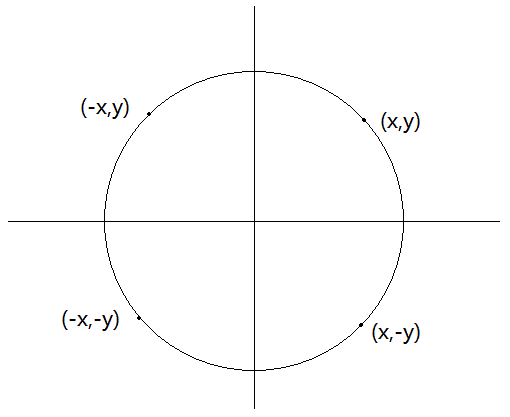假如我们确定了圆在第一象限的位置，则可以通过变换 y$y$ 的符号去生成圆在第二象限的位置。我们再对上述生成的全部位置进行相对于

x
$x$ 轴的符号变换就可以得到圆在第三四象限的位置。这就是四分法的基本思路。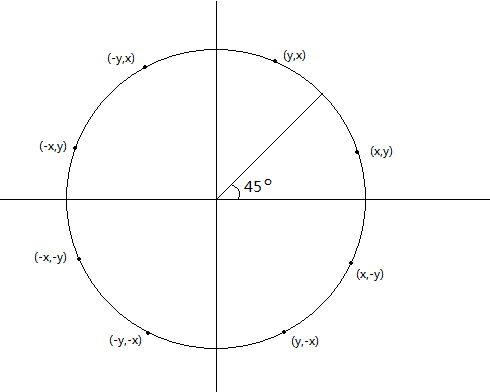在同一个象限内，如果按照 45o$45^o$ 进行分割，可以看出其坐标关于这个分割线是对称的。也就是在(0~45)度范围内的值可以通过简单变换映射到其他区域内。这种分割方式被称为圆的八分法。
1.2 勾股定理算法
在笛卡尔坐标系中，对于给定的原点

(xc,yc)
$(x_c,y_c)$和半径

r
$r$，圆上任意一点(x,y)$(x,y)$满足勾股定理

(x−xc)2+(y−yc)2=r2(1.2.1)

(x-x_c)^2+(y-y_c)^2=r^2 \tag{1.2.1}

利用这个算法我们可以通过任意

x
$x$ 值计算对应的 y$y$ 值

y=yc±r2−(xc−x)2‾‾‾‾‾‾‾‾‾‾‾‾‾√(1.2.2)

y=y_c \pm \sqrt{r^2-(x_c-x)^2} \tag{1.2.2}

现在我们通过示例程序演示该算法，仅展示思路，可使用任意语言或图形软件包实现。
void Pythagorean(int x,int y,int r){//使用勾股定理绘制圆
int start = x-r;
int end = r+x;
_IntArray _arr;
int size = 4*(end-start);
_arr = new IntArray(size);
IntArray_ arr = *_arr;
int r2 = r*r;
for(int i=start,j=0;i<end;i++){
int p = sqrt(float(r2-power(x-i)));
arr[j++]=i;
arr[j++]=y+p;
arr[j++]=i;
arr[j++]=y-p;
}
}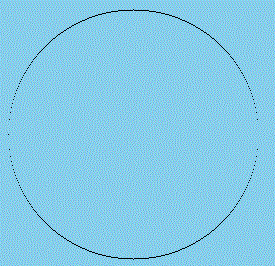通过结果 [图 1.2-1] 我们可以看出直接使用勾股定理会造成像素间距不一致的问题。处理方法有两种：第一种是在斜率的绝对值大于1后，交换

x
$x$和y$y$来调整间距；第二种方式是使用1.1节中提到的八分法。我们只需要计算八分之一的图形，剩下的操作就是简单映射即可。以下是使用八分法的示例
void Pythagorean(int xc,int yc,int r){//使用勾股定理和八分法绘制圆
int len = int(1+0.5*sqrt(2.0)*r);
int size = 16*len;
_IntArray _arr = new IntArray(size);
IntArray_ arr = *_arr;
int r2 = r*r;
int j=0;
for(int x=0;x<len;x++){
int p = sqrt(float(r2-power(x)));
arr[j++]=x;
arr[j++]=p;
}
int k=j;
for(int i=0;i<k;i+=2){
arr[j++] = arr[i+1];
arr[j++] = arr[i];
}
k = j;
for(int i=0;i<k;i+=2){
arr[j++] = arr[i];
arr[j++] = -arr[i+1];
}
k = j;
for(int i=0;i<k;i+=2){
arr[j++] = -arr[i];
arr[j++] = arr[i+1];
}
for(int i=0;i<j;i+=2){
arr[i]+=xc;
arr[i+1]+=yc;
}
}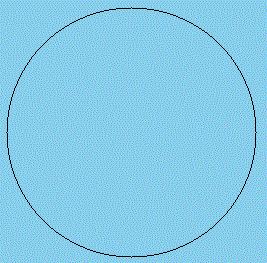在此示例中，我们先将圆计算时的圆心已原点(0，0)计算，在所有操作完成之后在平移到相应位置，这么做方便变换简化计算量。

总结：勾股定理算法简单，即使是使用八分法缩减运算规模，其大量复杂的开平方计算仍然是影响效率的关键因素。接下来，我们将介绍一种能够替换开平方运算的算法。

1.3 极坐标算法

极坐标系是一种常用的坐标系。其中坐标位置由到原点的极半径距离

r
$r$ 和距水平轴的角 θ$\theta$ 指定。正的角位移是逆时针的，而负的角位移是逆时针的。利用三角函数的定义，可以从极坐标系转换为笛卡尔坐标系。

x=rcosθ,y=rsinθ(1.3.1)

x=r cos\theta ,  y = r sin\theta \tag{1.3.1}

通过式(1.3.1)我们可以通过下列方程组表示圆方程

x=xc+rcosθ(1.3.2)

x=x_c+r cos\theta \tag{1.3.2}

y=yc+rsinθ(1.3.3)

y=y_c+r sin\theta \tag{1.3.3}

使用上述方式以单位角度为步长，可以在圆周上以等距离的点来绘制圆。
下面将使用程序展示极坐标算法的计算过程
    void Polar(int xc,int yc,int r){//使用极坐标系绘制圆
int point = 2;//每一度绘制两个点
double angle = 1.0/point;//每两个点之间的角度
int size = point*45*8*2;//使用八分法，
_IntArray _arr = new IntArray(size);
IntArray_ arr = *_arr;
int j=0;
for(int i=0;i<45;i++){
for(int m=0;m<point;m++){
arr[j++] = int(r*cos(((i+angle*m)*(PI/180))));//使用c库中的三角函数需要将角度转换为弧度
arr[j++] = int(r*sin(((i+angle*m)*(PI/180))));
}
}
int k=j;
for(int i=0;i<k;i+=2){
arr[j++] = arr[i+1];
arr[j++] = arr[i];
}
k = j;
for(int i=0;i<k;i+=2){
arr[j++] = arr[i];
arr[j++] = -arr[i+1];
}
k = j;
for(int i=0;i<k;i+=2){
arr[j++] = -arr[i];
arr[j++] = arr[i+1];
}
for(int i=0;i<j;i+=2){
arr[i]+=xc;
arr[i+1]+=yc;
}
}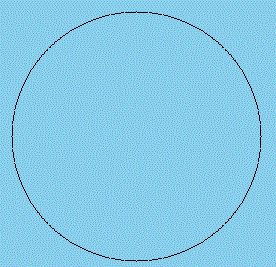总结：我们可以从图中看出其边缘有毛刺状突起，这是因为极坐标系运算以角度为步长而不是任何一个轴，这就导致其结果取整后不仅仅只会在一个方向上浮动。从效率的角度上说，虽然极坐标系统提供了等距离点，但是其三角函数计算仍然十分耗时。

1.4 中点圆算法

我们可以参照直线算法中的Bresenham算法，以决策参数的增量运算为基础，将圆的计算过程转换为简单的整数加减运算。

如同画线算法，我们在每一步中以单位间隔取样并确定离圆最近的像素位置。对于给定半径

r
$r$ 和屏幕中心 (xc,yc)$(x_c,y_c)$ ，可以现将圆的圆心放在坐标原点运算，在运算完成之后，再将圆移动到相应的位置。
为了应用中点圆算法，我们先定义一个圆函数

fcirc(x,y)=x2+y2−r2(1.4.1)

f_{circ}(x,y) = x^2+y^2-r^2 \tag{1.4.1}

任意一点

(x,y)
$(x,y)$ 均满足

f(n)=⎧⎩⎨⎪⎪<0,=0,>0,(x,y)在圆周边界之内(x,y)在圆周边界之上(x,y)在圆周边界之外(1.4.2)

f(n) =
\begin{cases}
\lt 0,  & \text{(x,y)在圆周边界之内} \\
=0, & \text{(x,y)在圆周边界之上} \\
\gt 0, & \text{(x,y)在圆周边界之外}
\end{cases} \tag{1.4.2}

我们需要使用(1.4.2)对每一个取样步上对接近圆周的两个像素的中点进行测试。因此，在中点算法中，圆函数(1.4.1)是决策参数。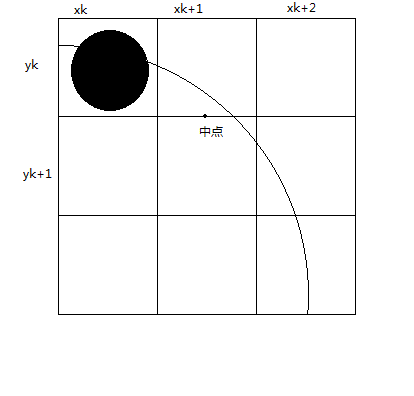图 [1.4-1] 给出了取样位置

xk+1
$x_k+1$ 上的中点，我们可以取出中点的坐标

(xk+1,(yk+yk−1)/2)
$(x_k+1,(y_k+y_k-1)/2)$ 也就是点

(xk+1,yk−12)
$(x_k+1,y_k-\frac{1}{2})$ ，接下来，我们只需要将中点位置代入方程(1.4.1)就可以算出决策参数

pk=fcirc(xk+1,yk−12)=(xk+1)2+(yk−12)2−r2(1.4.3)

p_k=f_{circ}(x_k+1,y_k-\frac{1}{2}) \\
=(x_k+1)^2+(y_k-\frac{1}{2})^2-r^2 \tag{1.4.3}

如果

pk<0
$p_k \lt 0$，那么这个圆的轨迹在中点的上方，所以我们选择扫描

yk
$y_k$ 这个像素，否则，我们扫描

yk−1
$y_k-1$这个像素。
接下来我们要寻找决策增量之间的关系。这使用了我们中学所学的数学归纳法。通过任意相邻两点之间的关系递推整个决策的关系。
通过式(1.4.4)我们可以求出下一个决策参数

pk=1=fcirc(xk+1+1,yk+1−12)=[(xk+1)+1]2+(yk+1−12)2−r2(1.4.4)

p_{k=1}=f_{circ}(x_{k+1}+1,y_{k+1}-\frac{1}{2}) \\
=[(x_k+1)+1]^2+(y_{k+1}-\frac{1}{2})^2-r^2 \tag{1.4.4}

我们可以找出相邻的两个决策参数之间的关系

pk+1=pk+2(xk+2)+(yk−yk+1)(1.4.5)

p_{k+1}=p_k+2(x_k+2)+(y_k-y_{k+1}) \tag{1.4.5}

其中

yk+1
$y_{k+1}$ 是

yk
$y_k$ 或者是

yk−1
$y_k-1$ 具体取值取决于

pk
$p_k$ 的符号。
我们已经计算出了决策增量的关系，接下来只需要计算出决策增量的初始值即可，对于圆心在坐标原点，半径为

r
$r$ 的圆来说，假设第一点从(0,r)$(0,r)$处起笔

p0=fcirc(1,r−12)=1+(r−12)2−r2=54−r(1.4.6)

p_0 = f_{circ}(1,r-\frac{1}{2}) \\
= 1+(r-\frac{1}{2})^2-r^2 \\
= \frac{5}{4}-r \tag{1.4.6}

如果为了简化运算，我们可以将决策参数近似为整数，而不用浮点数，其中

r
$r$ 也是整数

p0=1−r(1.4.7)p_0 = 1-r \tag{1.4.7}
接下来我们将使用一段代码来解释其流程：
    void Bresenham(int xc,int yc,int r){//使用中点圆算法
int j = 0;
int p = 1-r;//这里计算出的p0使用近似的整型来简化运算
int x = 0;
int y = r;
int size = 16*int(1+0.5*sqrt(2.0)*r);//整个运算过程会产生size/2个点
_IntArray _arr = new IntArray(size);
IntArray_ arr = *_arr;
for(int i=x;i<y;i++){
arr[j++] = x++;
if(p<0){//决策参数小于0说明中点在圆内，所以点要绘制在上方
arr[j++] = y;
p+=2*x+1;
}else{
arr[j++] = y--;
p+=2*x+1-2*y;
}
}
int k=j;
for(int i=0;i<k;i+=2){
arr[j++] = arr[i+1];
arr[j++] = arr[i];
}
k = j;
for(int i=0;i<k;i+=2){
arr[j++] = arr[i];
arr[j++] = -arr[i+1];
}
k = j;
for(int i=0;i<k;i+=2){
arr[j++] = -arr[i];
arr[j++] = arr[i+1];
}
for(int i=0;i<j;i+=2){
arr[i]+=xc;
arr[i+1]+=yc;
}
}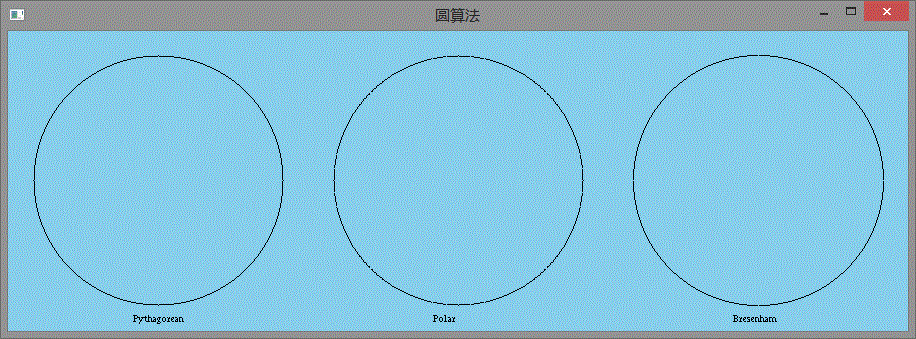图中示例分别为勾股定理(左)，极坐标系(中)和中点算法(右)

计算机图形学第四版.电子工业出版社.109~114 ↩


展开全文图形
• ## 区域生长算法

千次阅读 2015-08-26 23:08:14
算法介绍 区域生长，指将前景种子点扩展为更大区域的过程。...数据结构：栈，通过栈实现区域生长算法 数据：三维的病变视网膜图像 目的：分割视网膜图像中的病变区域，即色素上皮层脱离区域。实现过程
算法介绍  区域生长，指将前景种子点扩展为更大区域的过程。
预备知识  编程工具：VS2010， CMAKE  编程语言：C++  编程库：ITK  数据结构：栈，通过栈实现区域生长算法  数据：三维的病变视网膜图像  目的：分割视网膜图像中的病变区域，即色素上皮层脱离区域。
实现过程  （1） 读入原图像，前景种子点图像；  说明：前景种子点由形态学方法自动获取，也可以手动标注前景种子点。  （2） 定义区域生长的准则；  （3） 前景种子点入栈；对前景种子点逐个遍历，在当前种子点的26邻域内，如果有像素点满足生长条件，则该像素点入栈；直到栈为空，则算法结束；  （4） 添加约束条件，对区域生长结果进行限制；  （5） 将区域生长结果叠加在原图上，查看具体效果。
具体代码如下，并在VS2010上测试通过：
#include"itkImage.h"
#include"itkImageFileWriter.h"
#include<iostream>
#include<string>
#include <fstream>
#include <stack>
using namespace std;

int main(int argc, char* argv[])
{
char* srcImagePath = argv;
char* seedImagePath = argv;
char* dstImagePath = argv;
char* sur1Path = argv;//视网膜分层结果
char* sur12Path = argv;//视网膜分层结果

typedef itk::Image<unsigned short,3>InputImageType;
typedef itk::Image<unsigned short,3>OutputImageType;
typedef itk::Image<unsigned char,3>SeedImageType;
InputImageType::Pointer srcImage = InputImageType::New();
OutputImageType::Pointer dstImage = OutputImageType::New();
SeedImageType::Pointer seedImage = SeedImageType::New();

//允许种子点图像与源图像尺寸不同
InputImageType::SizeType imgSize = srcImage->GetLargestPossibleRegion().GetSize();
SeedImageType::SizeType seedImgSize = seedImage->GetLargestPossibleRegion().GetSize();

//结果图开辟内存
OutputImageType::IndexType index;
index=0;
index=0;
index=0;
OutputImageType::SizeType size;
size=imgSize;
size=imgSize;
size=imgSize;
OutputImageType::RegionType region;
region.SetIndex(index);
region.SetSize(size);
dstImage->SetRegions(region);
dstImage->Allocate();

struct seedpoint
{
int x;
int y;
int z;
};
stack<seedpoint>seedS;
seedpoint point;

//读入原数据
//unsigned short *srcData = new unsigned short[imgSize*imgSize*imgSize];
//通过GetBufferPointer()获取数据后，如果使用delete[] srcData 会导致程序崩溃
//所以不使用new开辟内存，借助与ITK内存管理机制来实现
const unsigned short* srcData  = srcImage->GetBufferPointer();//ITK数据转换为C++数据

//memset初始化结果图指针，第三个参数为内存单元大小（字节数）
unsigned short* resultData = new unsigned short[imgSize*imgSize*imgSize];
memset(resultData, 0, sizeof(unsigned short) * imgSize * imgSize * imgSize);

//前景种子点入栈
const unsigned char* seedData = seedImage->GetBufferPointer();//ITK数据转换为C++数据
for(int k=0; k < seedImgSize; k++)
for(int j = 0; j < seedImgSize; j++)
for(int i = 0; i < seedImgSize; i++)
{
if(seedData[k*seedImgSize*seedImgSize+j*seedImgSize+i] == 255)//255为前景灰度值
{
point.x = i;
point.y = j;
point.z = k;
seedS.push(point);
}
}

//初始化，将原图中所有像素点都标记为没有被遍历过
bool*flag = new bool[imgSize*imgSize*imgSize];
memset(flag, false, sizeof(bool) * imgSize * imgSize * imgSize);

//区域生长实现
unsigned short intensity = 13000;//前景平均灰度值
unsigned short threshold = 3000;//前景与背景灰度值差异

while(!seedS.empty())//栈为空则推出循环
{
seedpoint temppoint;
point=seedS.top();//取栈顶元素
seedS.pop();//元素弹栈
flag[point.z*imgSize*imgSize+point.y*imgSize+point.x] = true;//标记为已遍历
resultData[point.z*imgSize*imgSize+point.y*imgSize+point.x] = 255;//标记为亮区域

//图像边界出的像素点不进行处理
if((point.x >= 1) && (point.x <= (imgSize - 2)) &&
(point.y >= 1) && (point.y <= (imgSize - 2)) &&
(point.z >= 1) && (point.z <= (imgSize - 2)))
{
int x = point.x;
int y = point.y;
int z = point.z;

for(int i = -1; i <= 1; i++)
for(int j = -1; j <= 1; j++)
for(int k = -1; k <= 1; k++)
{
if(flag[(z + i) * imgSize * imgSize + (y + j) * imgSize + x + k] == false &&
srcData[(z + i) * imgSize * imgSize + (y + j) * imgSize + x + k] >= intensity - threshold &&
srcData[(z + i) * imgSize * imgSize + (y + j) * imgSize + x + k] <= intensity + threshold &&
(abs(srcData[(z + i) * imgSize * imgSize + (y + j) * imgSize + x + k] -
srcData[z * imgSize * imgSize + y * imgSize + x]) < threshold))
{
resultData[(z + i) * imgSize * imgSize + (y + j) * imgSize + x + k] = 255;//亮区域
temppoint.x = x + k;
temppoint.y = y + j;
temppoint.z = z + i;
seedS.push(temppoint);//新种子点入栈
flag[(z + i) * imgSize * imgSize + (y + j) * imgSize + x + k] = true;//标记为已遍历
}
}
}
}
delete[] flag;//用完后立即释放资源
flag = NULL;//避免野指针

//读入视网膜分层数据，对区域生长结果后处理
ifstream fin1, fin12;
int (*sur1) = new int;
int (*sur12) = new int;
fin1.open(sur1Path, ios::in);
fin12.open(sur12Path, ios::in);
for(int z = 0; z < 64; z++)
for(int x = 0; x < 512; x++)
{
fin1>>sur1[z][x];
fin12>>sur12[z][x];
}
fin1.close();
fin12.close();

//C++数据转ITK数据
OutputImageType::IndexType newvoxelIndex;
for(int z = 0; z < imgSize; ++z)
for(int y = 0; y < imgSize; ++y)
for(int x = 0; x < imgSize; ++x)
{
newvoxelIndex = x;
newvoxelIndex = y;
newvoxelIndex = z;
if(y < sur1[z][x] || y > sur12[z][x])//依据视网膜分层结果对区域生长结果进行约束
dstImage->SetPixel(newvoxelIndex, 0);
else
dstImage->SetPixel(newvoxelIndex, resultData[z*size*size+y*size+x]);
}
delete[] sur1;
sur1 = NULL;
delete[] sur12;
sur12 = NULL;
delete[] resultData;
resultData = NULL;

//输出结果图
typedef itk::ImageFileWriter<OutputImageType>WriterType;
WriterType::Pointer Writer = WriterType::New();
Writer->SetFileName(argv);
Writer->SetInput(dstImage);
Writer->Update();
return 0;
}

效果图如下，红色区域为区域生长结果。其中，半圆形区域为目标区域，其他为误分割区域。对于误分割区域，可以用形态学处理算法进行后处理。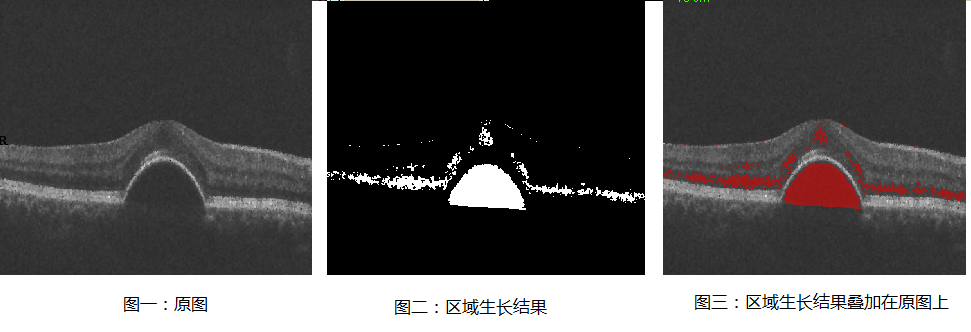展开全文图像分割 c++
• 目标：实现一个算法，模拟一个封闭二维区域圆形小球朝给定方向坠落的过程，实现二维区域的紧密填充。 像下面这样： 难点，及其简单解决： 1.如何把粒子移动尽可能远？ 图中的粒子i，能往下移动多远...
• 获取标记点近似成像中心及成像区域，成像区域内沿四个方向扫描得到像素级边缘点；利用灰度差重心法进行亚像素定位；对亚像素级边缘点进行椭圆拟合，得到标记点成像中心位置。实验结果表明，像素级边缘检测算法运行...
• 这类算法建立多边形边边界的图象形式数据之上，并还需提供多边形界一点的坐标。所以，它一般只能用于人机交互填色，而难以用于程序填色。 GIS中用于栅格填色 2、扫描线填色算法(Scan-Line Filling) 这类算法...
• 为求解矩形区域内圆形Packing 问题，提出一种启发式模拟退火算法。寻求多个圆一个矩形区域内的优良布局，使这些圆两两互不嵌入地放置。算法从任一初始构形出发，采用模拟退火(SA)算法进行全局寻优，SA 执行...
• 光栅显示平面上，多边形是封闭的，它是用某一边界色围成的一个闭合区域，填充是逐行进行的，即用扫描线逐行对多边形求交，交点对之间填充。边界标志填充算法就是逐行处理时，利用边界或边界颜色作为标志来...
• 方法一：引射线法 ...这是所有方法中计算量最小的方法，光线追踪算法中有大量的应用。 using System; using System.Collections.Generic; using System.Linq; using System.Text; using Syst...多边形
• 最近做东西的时候用到了百度地图，想判断出经纬度是否在一个圆形范围 我用下面这段代码来取得圆形的范围： function getSquareBounds(centerPoi,r){ var a = Math.sqrt(2) * r; //正方形边长 mPoi =...
• 本实验基于STM32F103RC+TFTLCD屏，旨在于LCD屏上显示一个实心圆形，然而众所周知，对于屏幕而言，我们只能操作各个像素点，因此，选择出最接近标准圆形的像素点就成了本次实验的主要目的，最终得到的圆形大概应该...
• 可以使用Marc Edwards的超椭圆方程，直接计算出圆角矩形和圆形的像素区域，并且可以使用参数调节形状的区域。  x,y 是坐标，5是弧度的程度越大越接近矩形，60是半径系数，shader中需要归一化转换。 利用此公式...unity 圆角 shader
• fzero_all：fzero的扩展，可以给定的时间间隔找到多个根fzero_delves：使用 Delves-Lyness 算法在圆形或矩形搜索区域中找到复杂的根matlab
• 主要为大家详细介绍了js实现固定区域内的不重叠随机圆，文中示例代码介绍的非常详细，具有一定的参考价值，感兴趣的小伙伴们可以参考一下
• 对于每一个样本，算法会计算一段小距离ε（epsilon）的圆中有多少个其他样本，这个圆形区域叫做样本的 ε-neighborhood 如果一个样本有最少 min_samples个样本 ε-neighborhood中（包括此样本本身），就认为...
• 不规则区域的填充算法 一、简单递归 利用Dfs实现简单递归填充。 核心代码： // 简单深度搜索填充 （四连通） void DfsFill(int x, int y) { if (x &amp;lt; 0 || y &amp;lt; 0 || x&amp;gt;23 |...
• 聚类分析聚类分析背景小故事初识聚类分析什么是聚类分析聚类分析的要求基于...基于代表对象基于层次的聚类算法概念和特点代表算法BIRCH算法CURE算法CHAMELEON算法基于密度的聚类算法概念和特点代表算法DBSCAN算法O...聚类分析
• K-means算法：例子中的问题，我们发现使用K-Means算法已经不再适用，因为K-means算法是基于距离度量的一种算法，K-means的理想状态就是聚完类后，每个点都能离所属簇的质心距离最近。（如果去掉外面的圈圈，这sklearn
• ## SIFT算法

万次阅读 多人点赞 2018-03-24 10:17:30
尺度不变特征转换(Scale-invariant feature transform或SIFT)是一种电脑视觉的算法用来侦测与描述影像中的局部性特征，它空间尺度中寻找极值点，并提取出其位置、尺度、旋转不变量，此算法由 David Lowe1999...特征点检测
• GeoUtils.js提供若干几何算法，用来帮助用户判断点与矩形、 圆形、多边形线、多边形面的关系,并提供计算折线长度和多边形的面积的公式。 主入口类是GeoUtils。 index.html中引入GeoUtils.js。 ...插件 百度地图 高德地图
• ## SURF算法

千次阅读 2018-10-28 10:02:50
SURF (Speeded Up Robust Features, 加速稳健特征) 是一个稳健的图像识别和描述算法，首先于2006年发表ECCV大会上。这个算法可被用于计算机视觉任务，如物件识别和3D重构。他部分的灵感来自于 SIFT 算法。SURF标.....加速稳健特征 机器视觉
• ## 算法

千次阅读 2006-11-13 17:10:00
A*寻路算法中，我们通过从点A开始，检查相邻方格的方式，向外扩展直到找到目标。 我们做如下操作开始搜索：  1，从点A开始，并且把它作为待处理点存入一个“开启列表”。开启列表就像一张购物清单。尽管现在列表...扩展 matrix
• ## 图像算法研究---背景虚化算法

万次阅读 热门讨论 2017-07-24 20:18:31
本文抠图算法基础上，实现了一种如何实现单反背景虚化效果的算法，更具单反的真实感，跟大家分享一下！背景虚化
• 算法采用传统的检测方法实现，通过对仪表的观察和分析，发现仪表的指针特征较为明显，且仪表形状为圆形，故算法决定采用Hough直线检测方法检测指针的位置，采用Hough检测圆的方法实现对仪表的检测。 文章目录算法的...计算机视觉 机器学习 经验分享 人工智能
• Web前端面试指导 四十二 如何页面上实现一个圆形的可点击区域...